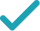Type a math problemSolve for xSteps by Finding Square Root
Steps by Finding Square Root
Variable cannot be equal to since division by zero is not defined. Multiply both sides of the equation by , the least common multiple of .
Cancel out and .
Multiply and to get .
Multiply and to get .
Combine and to get .
Multiply and to get .
Divide both sides by .
Divide by to get .
Take the square root of both sides of the equation.
GraphGraph Both Sides in 2D
Graph in 2DGiving is as easy as 1, 2, 3
Get 1,000 points to donate to a school of your choice when you join Give With Bing
\frac{1}{2}x\times 2x+2xx=2\times 51
Variable x cannot be equal to 0 since division by zero is not defined. Multiply both sides of the equation by 2x, the least common multiple of 2,x.
xx+2xx=2\times 51
Cancel out 2 and 2.
x^{2}+2xx=2\times 51
Multiply x and x to get x^{2}.
x^{2}+2x^{2}=2\times 51
Multiply x and x to get x^{2}.
3x^{2}=2\times 51
Combine x^{2} and 2x^{2} to get 3x^{2}.
3x^{2}=102
Multiply 2 and 51 to get 102.
x^{2}=\frac{102}{3}
Divide both sides by 3.
x^{2}=34
Divide 102 by 3 to get 34.
x=\sqrt{34} x=-\sqrt{34}
Take the square root of both sides of the equation.
\frac{1}{2}x\times 2x+2xx=2\times 51
Variable x cannot be equal to 0 since division by zero is not defined. Multiply both sides of the equation by 2x, the least common multiple of 2,x.
xx+2xx=2\times 51
Cancel out 2 and 2.
x^{2}+2xx=2\times 51
Multiply x and x to get x^{2}.
x^{2}+2x^{2}=2\times 51
Multiply x and x to get x^{2}.
3x^{2}=2\times 51
Combine x^{2} and 2x^{2} to get 3x^{2}.
3x^{2}=102
Multiply 2 and 51 to get 102.
3x^{2}-102=0
Subtract 102 from both sides.
x=\frac{0±\sqrt{0^{2}-4\times 3\left(-102\right)}}{2\times 3}
This equation is in standard form: ax^{2}+bx+c=0. Substitute 3 for a, 0 for b, and -102 for c in the quadratic formula, \frac{-b±\sqrt{b^{2}-4ac}}{2a}.
x=\frac{0±\sqrt{-4\times 3\left(-102\right)}}{2\times 3}
Square 0.
x=\frac{0±\sqrt{-12\left(-102\right)}}{2\times 3}
Multiply -4 times 3.
x=\frac{0±\sqrt{1224}}{2\times 3}
Multiply -12 times -102.
x=\frac{0±6\sqrt{34}}{2\times 3}
Take the square root of 1224.
x=\frac{0±6\sqrt{34}}{6}
Multiply 2 times 3.
x=\sqrt{34}
Now solve the equation x=\frac{0±6\sqrt{34}}{6} when ± is plus.
x=-\sqrt{34}
Now solve the equation x=\frac{0±6\sqrt{34}}{6} when ± is minus.
x=\sqrt{34} x=-\sqrt{34}
The equation is now solved.